# QuTiP: Independent verification of Q-CTRL optimization solutions

Using QuTiP to simulate controls optimized with BOULDER OPAL

BOULDER OPAL includes functionality for creating optimized control pulses and simulating quantum systems. Performing simulations of optimized control pulses can then provide additional information about the properties of the controls, such as noise robustness. Another use for simulation is to verify that the optimized controls perform as well as predicted by the optimizer. For this purpose, using an entirely separate, independent tool provides a more meaningful validation. In this notebook, we use the QuTiP library to verify the performance of an optimized Q-CTRL pulse implementing a robust $X$ gate.

## Imports and initialization

All usage of the BOULDER OPAL begins by importing the qctrl package and starting a session.

# Essential imports
import numpy as np
from qctrl import Qctrl

# QuTiP imports
import qutip

# Predefined pulse imports
from qctrlopencontrols import new_primitive_control

# Plotting imports
import matplotlib.pyplot as plt
from matplotlib import cm
from qctrlvisualizer import plot_controls

# Starting a session with the API
qctrl = Qctrl()


## Creating an optimized pulse

As an example, we will consider a single-qubit $X$ gate in a system obeying the following time-dependent Hamiltonian:

$$H(t) = \frac{1 + \beta_\Omega (t)}{2} \left[ \Omega(t) \sigma_- + \Omega^* (t) \sigma_+ \right] + \frac{1}{2} \Delta(t) \sigma_z + \frac{1}{2} \eta(t) \sigma_z,$$

where the Rabi frequency $\Omega(t)$ and the detuning $\Delta(t)$ are the two controls that will be optimized. These controls will be limited by a constant upper bound to their modulus, $\Delta_\mathrm{max}=\Omega_\mathrm{max}$, which we are assuming to be the same, for simplicity.

The functions $\beta_\Omega(t)$ and $\eta(t)$ represent multiplicative and additive noises, respectively, against which we will optimize our pulses.

### Obtaining robust controls

To set up a system using BOULDER OPAL, you can follow the instructions in the Setting up quantum systems user guide. In this example, we will create two versions of this system: one undergoing a robust optimized pulse, and another one just undergoing the primitive $X$ gate. These two systems will be used to compare the robustness of the two pulses. Details of how a robust optimized pulse can be calculated are found in the Optimization user guide.

# Defining system constants
omega_max = (2.*np.pi) * 2.e6 # Hz
delta_max = omega_max
gate_duration = 1.0e-6 # s

# Defining optimization parameters
number_of_segments = 256

# Standard matrices
identity = np.identity(2, dtype=np.complex)
sigma_x = np.array([[0., 1.], [1., 0.]], dtype=np.complex)
sigma_z = np.array([[1., 0.], [0., -1.]], dtype=np.complex)
sigma_m = np.array([[0., 1.], [0., 0.]], dtype=np.complex)

# List of types of pulses to be tested and compared
schemes = {'primitive': {},
'robust': {}}

for scheme, saved_objects in schemes.items():
# Defining the system
saved_objects['system'] = qctrl.factories.systems.create(
name=scheme,
hilbert_space_dimension=2
)

# Defining controls
saved_objects['drive'] = qctrl.factories.drive_controls.create(
name=r'$\Omega$',
operator=.5*sigma_m,
system=saved_objects['system'],
)

saved_objects['shift'] = qctrl.factories.shift_controls.create(
name=r'$\Delta$',
operator=.5*sigma_z,
system=saved_objects['system'],
)

# Defining noises
saved_objects['amplitude_noise'] = qctrl.factories.control_noises.create(
control=saved_objects['drive'],
name='Amplitude noise',
)

name='Dephasing noise',
operator=.5*sigma_z,
system=saved_objects['system'],
)

# Defining primitive pulse
pulse = new_primitive_control(
rabi_rotation=np.pi,
azimuthal_angle=0.,
maximum_rabi_rate=omega_max,
name='primitive',
)

qctrl.factories.custom_pulses.create(
control=schemes['primitive']['drive'],
segments=[{'duration': d, 'value': v}
for d, v in zip(pulse.durations,
pulse.rabi_rates * np.exp(1.j*pulse.azimuthal_angles))],
)

qctrl.factories.custom_pulses.create(
control=schemes['primitive']['shift'],
segments=[{'duration': d, 'value': v}
for d, v in zip(pulse.durations, pulse.detunings)],
)

schemes['primitive']['duration'] = np.cumsum(pulse.durations)

# Defining robust pulses
qctrl.factories.optimum_pulses.create(
control=schemes['robust']['drive'],
duration=gate_duration,
fixed_modulus=False,
segment_count=number_of_segments,
upper_bound=omega_max,
maximum_slew_rate=omega_max/5.,
)

qctrl.factories.optimum_pulses.create(
control=schemes['robust']['shift'],
duration=gate_duration,
fixed_modulus=False,
segment_count=number_of_segments,
upper_bound=delta_max,
maximum_slew_rate=delta_max/10.,
)

schemes['robust']['duration'] = gate_duration

# Defining the target
for saved_objects in schemes.values():
qctrl.factories.targets.create(
projection_operator=identity,
system=saved_objects['system'],
unitary_operator=sigma_x,
)

# Calculating the robust pulse
qctrl.services.robust_optimization.run(system=schemes['robust']['system'])

for scheme in schemes.values():
scheme['system'].refresh()

100%|██████████| 23/23 [04:18<00:00, 11.26s/it, running=0]

# Plotting controls using qctrlvisualizer
for scheme, saved_objects in schemes.items():
saved_objects['controls'] = {control.name: control.pulse.segments
for control in saved_objects['system'].controls
if hasattr(control.pulse, 'segments')}

fig = plt.figure()
fig.suptitle(scheme.capitalize() + " controls")
plot_controls(fig, saved_objects['controls'])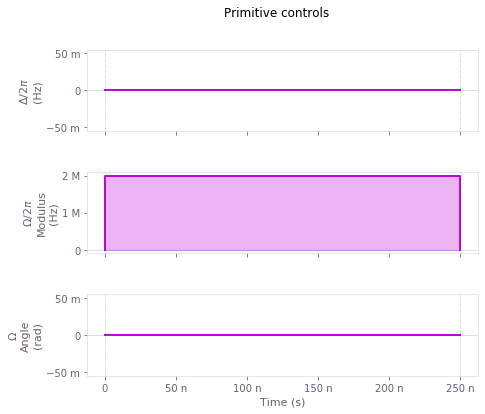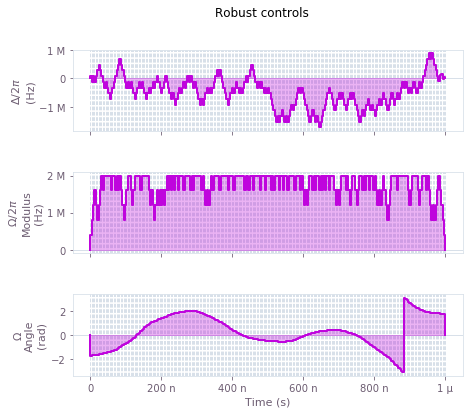Above we show a visual representation of what the controls look like, using qctrlvisualizer package. Here, $\Omega(t)$ represents the Rabi rate and $\Delta(t)$ is the detuning, according to the Hamiltonian:

$$H(t) = \frac{1 + \beta_\Omega (t)}{2} \left[ \Omega(t) \sigma_- + \Omega^* (t) \sigma_+ \right] + \frac{1}{2} \Delta(t) \sigma_z + \frac{1}{2} \eta(t) \sigma_z.$$

## Independent verification with QuTiP

QuTiP is an open-source library for simulating open quantum systems in Python. We will use it to simulate the evolution of a system undergoing our optimized pulse. By calculating the fidelity of the gate at the end of the simulation, we are able to characterize the robustness of the gate against different kinds of errors. As QuTiP has tools to perform the simulation of open systems, we will use it to characterize our pulses in the presence of a loss process. We will also compare their scans of quasi-static noise with those generated with BOULDER OPAL for the case of non-Markovian noise, to check that they match.

### Converting the controls into QuTiP format and simulating them

The first step to perform the independent verification is to make sure that QuTiP can understand the controls that we are applying to the system. Once these controls have been adapted into QuTiP format, we can simulate their evolution using both packages, and compare the results. Starting with a qubit initially in the state $\left| 0 \right\rangle$, the expected evolution with the $X$ gate is to flip it to $\left| 1 \right\rangle$.

By setting the noise functions to zero in the system Hamiltonian, we obtain the evolution of the pulses in the ideal case. Both the BOULDER OPAL and QuTiP are capable of calculating these noiseless simulations, and a comparison of them is a first test of whether the pulses are being treated the same by both packages.

# Transforming the piecewise constant controls into functions of time
segment = lambda segments, duration, t: min(segments-1, int(np.floor(segments*t/duration)))
Delta = lambda controls, duration, t, args: controls[r'$\Delta$'][segment(len(controls[r'$\Delta$']),
duration, t)]['value']
Omega = lambda controls, duration, t, args: controls[r'$\Omega$'][segment(len(controls[r'$\Omega$']),
duration, t)]['value']

# Defining the time-dependent Hamiltonian in a a format that QuTiP can parse
for scheme, saved_objects in schemes.items():
saved_objects['H'] = [[.5*qutip.sigmam(), (lambda t, args: Omega(saved_objects['controls'],
saved_objects['duration'], t, args))],
[.5*qutip.sigmap(), (lambda t, args: Omega(saved_objects['controls'],
saved_objects['duration'], t, args).conj())],
[.5*qutip.sigmaz(), (lambda t, args: Delta(saved_objects['controls'],
saved_objects['duration'], t, args))]]

# Defining initial state and range of times
ket_0 = np.array([1., 0.], dtype=np.complex)
point_times = np.linspace(0., gate_duration, 101)

# Defining and calculating simulation with BOULDER OPAL
for saved_objects in schemes.values():
qctrl.factories.coherent_simulations.create(
initial_state_vector=ket_0,
point_times=point_times[point_times <= saved_objects['duration']],
system=saved_objects['system'],
)

qctrl.services.coherent_simulations.run(saved_objects['system'])

saved_objects['system'].refresh()

100%|██████████| 2/2 [00:11<00:00,  5.58s/it, running=0]
100%|██████████| 2/2 [00:10<00:00,  5.38s/it, running=0]

qutip_ket_0 = qutip.basis(2, 0)
qutip_ket_1 = qutip.basis(2, 1)

# Calculating the coherent evolutions using QuTiP
qutip_noiseless_simulations = {}
for scheme, saved_objects in schemes.items():
qutip_noiseless_simulations[scheme] = qutip.sesolve(
saved_objects['H'], qutip_ket_0, point_times[point_times <= saved_objects['duration']], [])

for scheme, saved_objects in schemes.items():
population_0 = [np.abs(frame['state_vector'])**2.
for frame in saved_objects['system'].simulations.trajectories.frames]
population_1 = [np.abs(frame['state_vector'])**2.
for frame in saved_objects['system'].simulations.trajectories.frames]

qutip_population_0 = [np.abs(ket)**2.
for ket in qutip_noiseless_simulations[scheme].states]
qutip_population_1 = [np.abs(ket)**2.
for ket in qutip_noiseless_simulations[scheme].states]

fig = plt.figure()
fig.suptitle(scheme.capitalize() + " controls")
plt.plot(point_times[point_times <= saved_objects['duration']]/1e-6,
qutip_population_0, label="Population |0> (QuTiP)", color="#BF04DC", alpha=0.6)
plt.plot(point_times[point_times <= saved_objects['duration']]/1e-6,
qutip_population_1, label="Population |1> (QuTiP)", color="#680CE9", alpha=0.6)
plt.plot(point_times[point_times <= saved_objects['duration']]/1e-6,
population_0, ':', label="Population |0> (Q-CTRL)", color="#BF04DC")
plt.plot(point_times[point_times <= saved_objects['duration']]/1e-6,
population_1, ':', label="Population |1> (Q-CTRL)", color="#680CE9")
plt.xlim(0., saved_objects['duration']/1e-6)
plt.ylim(0., 1.)
plt.xlabel("Time ($\\mu$s)")
plt.ylabel("Probability")
plt.legend(loc=3, bbox_to_anchor=(1., 0.7))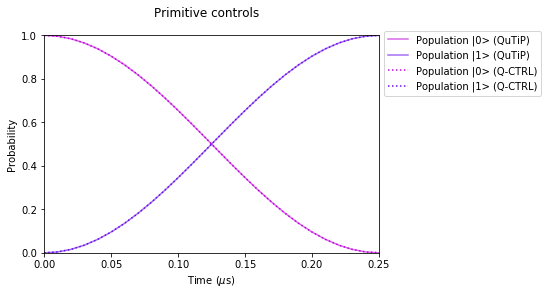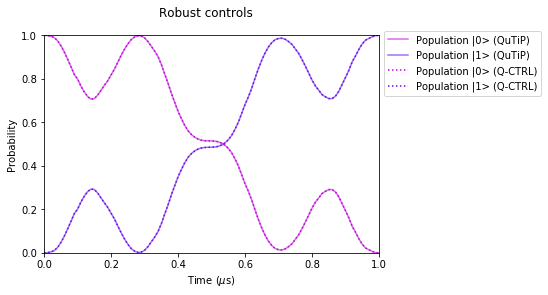The graphs above show the evolution of the state populations during the primitive and the robust X gate. Both reach the same final state in this noiseless simulation, but the robust case has a more intricate trajectory. The simulations obtained via the BOULDER OPAL (dotted) and QuTiP (solid) are so close to the point of being indistinguishable.

### Comparing quasi-static scans of amplitude errors

A quasi-static scan can help us characterize the robustness of a set of controls against a particular kind of noise. To obtain them, we give a constant value to the noise throughout the application of the gate, and then observe how varying this static value affects the final infidelity of the system. In the case of amplitude errors, our quasi-static scan will consist in varying a parameter that multiplies the Rabi frequency of the system.

BOULDER OPAL provides built-in functions that calculate this, as detailed in the Quasi-static scans user guide. For QuTiP, we will manually provide Hamiltonians with each of the static values of the noise parameter, and calculate the final infidelity. As we are interested in the operational fidelity, in QuTiP we will calculate the evolution of two qubits in a Bell state, only one of which evolves according to the Hamiltonian, and then calculate their final state fidelity. BOULDER OPAL already outputs the results in terms of the operational infidelity, so the intermediary step of calculating the entanglement infidelity is not necessary.

# Range of the scan
beta_values = np.linspace(-.5, .5, 101)

# Calculating the scan with BOULDER OPAL
for saved_objects in schemes.values():
amplitude_profile = qctrl.factories.quasi_static_functions.create(
x_noise=saved_objects['amplitude_noise'],
x_coefficients=beta_values,
)

beta_scan = qctrl.services.quasi_static_functions.calculate(
amplitude_profile)

saved_objects['beta_infidelities'] = [point['infidelity']
for point in beta_scan.sampled_points]

100%|██████████| 2/2 [00:11<00:00,  5.89s/it, running=1]
100%|██████████| 2/2 [00:22<00:00, 11.11s/it, running=1]

# Defining the Bell state and its ideal evolution
bell_state = qutip.bell_state()
ideal_final_state = qutip.tensor(
qutip.sigmax(), qutip.identity(2)) * bell_state

# Calculating the scan with QuTiP
for saved_objects in schemes.values():
saved_objects['qutip_beta_infidelities'] = []
for beta in beta_values:
Hamiltonian = [[.5*(1.+beta)*qutip.sigmam(),
(lambda t, args: Omega(saved_objects['controls'],
saved_objects['duration'], t, args))],
[.5*(1.+beta)*qutip.sigmap(),
(lambda t, args: Omega(saved_objects['controls'],
saved_objects['duration'], t, args).conj())],
[.5*qutip.sigmaz(),
(lambda t, args: Delta(saved_objects['controls'],
saved_objects['duration'], t, args))]]

for term in Hamiltonian:
term = qutip.tensor(term, qutip.identity(2))

qutip_simulation = qutip.sesolve(
Hamiltonian, bell_state, point_times[point_times <= saved_objects['duration']], [])
qutip_final_state = qutip_simulation.states[-1]

saved_objects['qutip_beta_infidelities'].append(
1.-qutip.fidelity(ideal_final_state, qutip_final_state)**2.)

# Plotting the results
for scheme, saved_objects in schemes.items():
fig = plt.figure()
fig.suptitle(scheme.capitalize() + " controls")
plt.xlabel(r"Static amplitude noise, $\beta_\Omega$")
plt.ylabel("Infidelity")
plt.xlim(-.5, .5)
plt.ylim(0., .5)
plt.plot(beta_values, saved_objects['qutip_beta_infidelities'], label="QuTiP", color="#BF04DC")
plt.plot(beta_values, saved_objects['beta_infidelities'], ':', label="Q-CTRL", color="#680CE9")
plt.legend()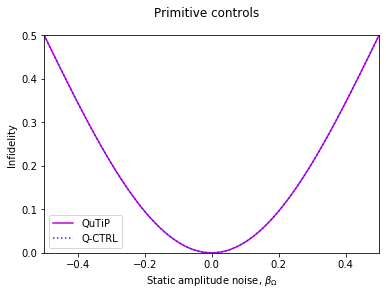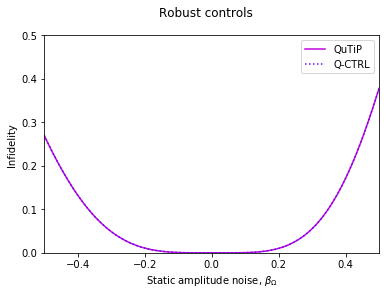In the graphs above we can see how a static amplitude noise represented by $\beta_\Omega$ can affect the fidelity of the system. This parameter $\beta_\Omega$ represents a multiplicative error in the amplitude of $\Omega(t)$, as we had defined in the Hamiltonian of the system:

$$H(t) = \frac{1 + \beta_\Omega}{2} \left[ \Omega(t) \sigma_- + \Omega^* (t) \sigma_+ \right] + \frac{1}{2} \Delta(t) \sigma_z.$$

Notice that for the purposes of this robustness characterization we are not taking into account dephasing noise.

The quasi-static scan of the robust pulse presents a much flatter profile around the origin than the primitive pulse, showing that the robust controls are less sensitive to amplitude noise. We also see that quasi-static scans obtained by QuTiP and BOULDER OPAL.

### Comparing quasi-static scans of dephasing noise

The procedure for generating a quasi-static scan for dephasing noise is similar to the process we used above for amplitude errors, the difference being that now we will add a static term containing $\sigma_z$ to the Hamiltonian, instead of changing a multiplicative factor. Once again, we can compare the robustness of the optimized pulse against the primitive, and also compare the results obtained via BOULDER OPAL with those from QuTiP.

# Range of the scan
eta_values = delta_max*np.linspace(-.5, .5, 101)

# Defining and calculating the scan
for saved_objects in schemes.values():
dephasing_profile = qctrl.factories.quasi_static_functions.create(
x_noise=saved_objects['dephasing_noise'],
x_coefficients=eta_values,
)

eta_scan = qctrl.services.quasi_static_functions.calculate(dephasing_profile)

saved_objects['eta_infidelities'] = [point['infidelity'] for point in eta_scan.sampled_points]

100%|██████████| 2/2 [00:17<00:00,  8.86s/it, running=1]
100%|██████████| 2/2 [00:27<00:00, 13.92s/it, running=0]

# Calculating the scan with QuTiP
for saved_objects in schemes.values():
saved_objects['qutip_eta_infidelities'] = []
for eta in eta_values:
Hamiltonian = ([[qutip.tensor(term, qutip.identity(2)), term]
for term in saved_objects['H']]
+[.5*eta*qutip.tensor(qutip.sigmaz(), qutip.identity(2))])

qutip_simulation = qutip.sesolve(
Hamiltonian, bell_state, point_times[point_times <= saved_objects['duration']], [])
qutip_final_state = qutip_simulation.states[-1]

saved_objects['qutip_eta_infidelities'].append(1.-qutip.fidelity(ideal_final_state, qutip_final_state)**2.)

# Plotting the results
for scheme, saved_objects in schemes.items():
fig = plt.figure()
fig.suptitle(scheme.capitalize() + " controls")
plt.xlabel(r"Static dephasing noise, $\eta/\Delta_\mathrm{max}$")
plt.ylabel("Infidelity")
plt.xlim(-.5, .5)
plt.ylim(0., .5)
plt.plot(eta_values/delta_max, saved_objects['qutip_eta_infidelities'], color="#BF04DC", label="QuTiP")
plt.plot(eta_values/delta_max, saved_objects['eta_infidelities'], ':', color="#680CE9", label="Q-CTRL")
plt.legend()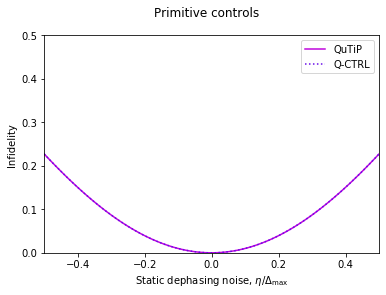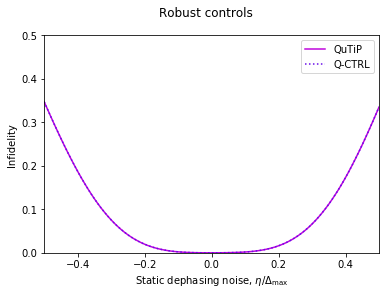In the graphs above, we are seeing the infidelity of the primitive and optimized controls at the end of the gate, when subjected to static dephasing noise represented by the parameter $\eta$, as defined in the Hamiltonian:

$$H(t) = \frac{1}{2} \left[ \Omega(t) \sigma_- + \Omega^* (t) \sigma_+ \right] + \frac{1}{2} \Delta(t) \sigma_z + \frac{1}{2} \eta \sigma_z.$$

Notice that for the purposes of this characterization we are assuming there is no amplitude noise.

Here we once again see a flatter profile of the quasi-static scan around the origin for the case of the optimized pulse, representing less sensitivity to dephasing noise. Again, the results obtained with QuTiP and BOULDER OPAL are indistinguishably close.

### Obtaining a quasi-static scan of dephasing noise with a loss process

Besides these static coherent noises, QuTiP also allows us to calculate the evolution of an open system. In particular, we can model the same quasi-static evolution used in the dephasing scans above, but this time including a finite value for the time $T_1$, which characterizes a simultaneous loss process.

These open-system dynamics can be simulated in QuTiP as the solution for a Lindblad equation, in which we include extra Lindblad operators $L_i$ to the original Schrödinger equation:

$$\frac{\mathrm{d}}{\mathrm{d}t} \rho(t) = - i \left[ H(t), \rho(t) \right] + \sum_i \left[ L_i \rho(t) L_i^\dagger - \frac{1}{2} \left\{ \rho(t), L_i^\dagger L_i \right\} \right].$$

Above, $\rho(t)$ is the density matrix and $H(t)$ is the original Hamiltonian.

For the loss process, the Lindblad operator to be included is $\sigma_-/\sqrt{T_1}$, which, in isolation, reduces the population in the $\left| 0 \right\rangle$ state by $e^{-t/T_1}$. Its joint dynamics together with a static term representing the dephasing process will be simulated below with QuTiP, the results then used to generate quasi-static scans that represent a situation with both finite $T_1$ and $T_2$.

In this simulation, $T_1$ will be represented by a Markovian loss process, while $T_2$ will be represented by a static detuning in place of a non-Markovian dephasing noise. We choose our $T_1$ as being 100 times longer than our robust pulse, which means $T_1 = 100 \mu \mathrm{s}$. This number is of the same order of magnitude as the $T_1$ in current superconducting devices.

t1 = 100*gate_duration

# Using QuTiP to calculate the scan
for saved_objects in schemes.values():
saved_objects['qutip_eta_t1_infidelities'] = []
for eta in eta_values:

Hamiltonian = ([[qutip.tensor(term, qutip.identity(2)), term]
for term in saved_objects['H']]
+[.5*eta*qutip.tensor(qutip.sigmaz(), qutip.identity(2))])

qutip_simulation = qutip.mesolve(
Hamiltonian, bell_state, point_times[point_times <= saved_objects['duration']], lindbladian)
qutip_final_state = qutip_simulation.states[-1]

saved_objects['qutip_eta_t1_infidelities'].append(
1.-qutip.fidelity(ideal_final_state, qutip_final_state)**2.)

# Plotting the results
for scheme, saved_objects in schemes.items():
fig = plt.figure()
fig.suptitle(scheme.capitalize() + " controls")
plt.xlabel(r"Static dephasing noise ($\eta/\Delta_\mathrm{max}$)")
plt.ylabel("Infidelity")
plt.xlim(-.5, .5)
plt.ylim(0., .5)
plt.plot(eta_values/delta_max, saved_objects['qutip_eta_t1_infidelities'], color="#BF04DC")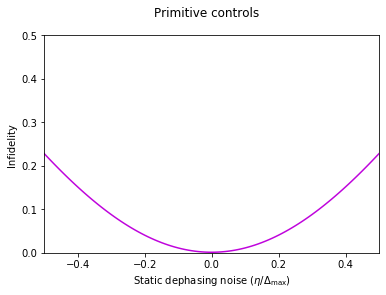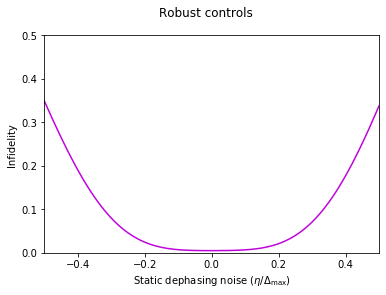In the graphs above, we perform a scan of static values of $\eta$, which are constant detuning terms added to the Lindblad equation:

$$\frac{\mathrm{d}}{\mathrm{d}t} \rho(t) = - i \left[ \frac{1}{2} \left[ \Omega(t) \sigma_- + \Omega^* (t) \sigma_+ \right] + \frac{1}{2} \Delta(t) \sigma_z + \frac{1}{2} \eta \sigma_z, \rho(t) \right] + \frac{1}{T_1} \left[ \sigma_- \rho(t) \sigma_+ - \frac{1}{2} \left\{ \rho(t), \sigma_+ \sigma_- \right\} \right],$$

As we are only interested in errors caused by the finite-time $T_1$ and $T_2$, we will be ignoring the effect of amplitude noise. The loss process, however, is still present in the form of the Lindbladian term in the equation above.

The graphs above still show a flatter surface for the robust controls, when compared to the primitive pulse. However, the presence of the loss process causes the robust curve to be shifted up, due to the fact that the robust pulse is four times longer than the primitive one.

### Obtaining simultaneous scans of dephasing noise and a loss process

Once we have the ability to include both dephasing terms and a loss process in our simulations, we can perform scans of the sensitivity of our noise to these two sources of error. Now, we go beyond the previous section, where we only changed the static dephasing term that represents $T_2$, and also vary the value of $T_1$.

# Using QuTiP to calculate the scan
t1_values = np.linspace(1*gate_duration, 500*gate_duration, 101)

for saved_objects in schemes.values():
saved_objects['qutip_t1_t2_infidelities'] = []
for t1 in t1_values:
saved_objects['qutip_t1_t2_infidelities'].append([])
for eta in eta_values:

Hamiltonian = ([[qutip.tensor(term, qutip.identity(2)), term]
for term in saved_objects['H']]
+ [.5*eta*qutip.tensor(qutip.sigmaz(), qutip.identity(2))])

qutip_simulation = qutip.mesolve(
Hamiltonian, bell_state, point_times[point_times <= saved_objects['duration']], lindbladian)
qutip_final_state = qutip_simulation.states[-1]

saved_objects['qutip_t1_t2_infidelities'][-1].append(
1.-qutip.fidelity(ideal_final_state, qutip_final_state)**2.)

# Plotting the results
X, Y = np.meshgrid(np.arange(len(eta_values)), np.arange(len(t1_values)))

for scheme, saved_objects in schemes.items():
Z = np.array(saved_objects['qutip_t1_t2_infidelities'])
fig = plt.figure(figsize=(7, 5))
plt.title(scheme.capitalize() + " controls")
contours = plt.contour(X, Y, Z, levels=[0.005, .01, .05, .1], colors='#680CEA')
plt.clabel(contours, inline=True, fontsize=8)
cmap_reversed = plt.cm.get_cmap('gray').reversed()
plt.imshow(Z, cmap=cmap_reversed, vmin=0, vmax=0.5)
plt.xticks(np.linspace(0, len(eta_values), 5),
[str(np.around(value, 2))
for value in np.linspace(min(eta_values/omega_max), max(eta_values/omega_max), 5)])
length = len(t1_values)
plt.yticks(np.concatenate((np.array(), np.linspace(length/5, length, 5)-1./length)),
['1.0'] + [str(np.around(value, 0))
for value in np.linspace(max(t1_values/1e-6)/5, max(t1_values/1e-6), 5)])
plt.xlabel(r"Static dephasing, $\eta/\Omega_\mathrm{max}$")
plt.ylabel(r"$T_1$ ($\mu$s)")
cbar = plt.colorbar()
cbar.set_ticks(np.linspace(0, 0.5, 6))
cbar.set_ticklabels(['0', '0.1', '0.2', '0.3', '0.4', '0.5'])
cbar.set_label('Infidelity')
plt.tight_layout()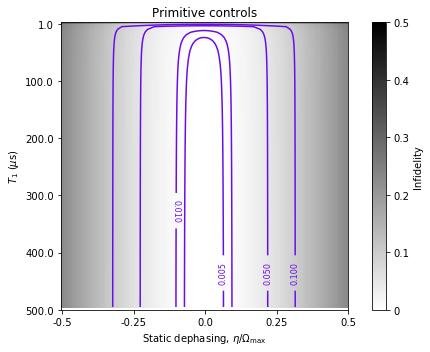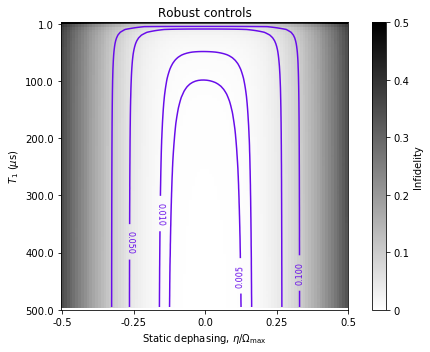The heat maps above show how the infidelity at the end of the gate changes when we vary a static dephasing term (related to the $T_2$ noise) and the loss rate (the inverse of $T_1$). Auxiliary contours in purple show where specific values of the infidelity occur.

We see again that, for a fixed value of $T_1$, the optimized controls present a wider region around the origin where the infidelity remains low, showing that they are more robust against dephasing noise. The longer duration of the robust pulse also makes it more sensitive to a decrease in $T_1$, which shifts further up the base value of its infidelity. This is visible in how the contour lines at the top of the graph close at a lower point for the robust controls than for the primitive ones.